# Semantic modeling of data science code¶

Evan Patterson
Stanford University, Statistics Department

Ioana Baldini, Aleksandra Mojsilović, Kush R. Varshney
IBM Research AI

PyData NYC
November 4, 2019

## Communicating data analysis...¶

...to people, with varying backgrounds, professional roles, and levels of expertise

...to machines, for the sake of:

• Summarization or visualization
• Meta-analysis or meta-learning
• Mining the scientific literature

Not independent: Machine understanding is the means, human understanding is the end.

## Communicating data analysis, the traditional way¶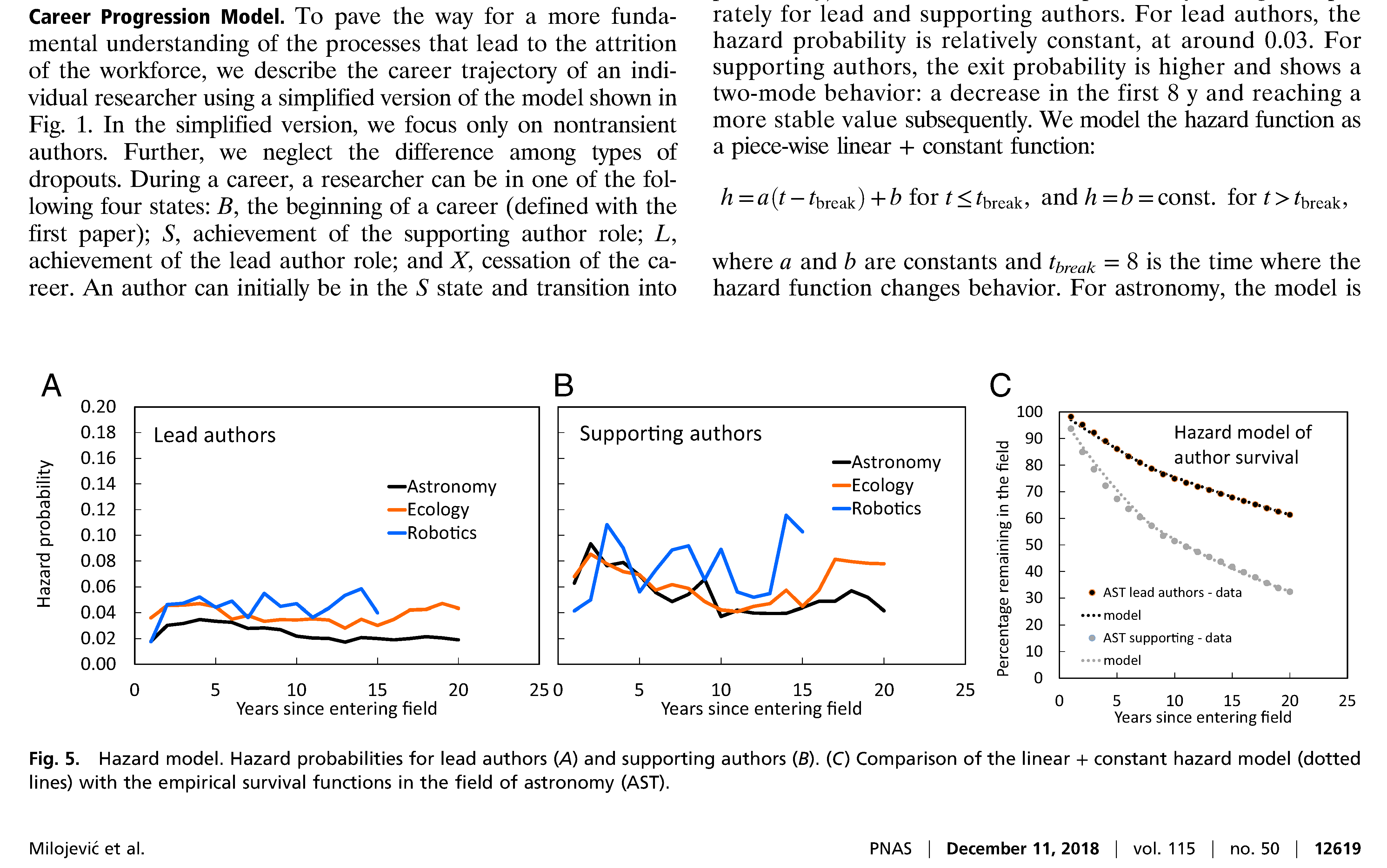## Communicating data analysis, the traditional way¶

Benefits of the traditional scientific report:

• Optimized for readability by humans

Problems:

• Model explorability is severely limited
• Important details may be omitted, hindering reproducibility
• Machine processing is extremely difficult

## Communicating data analysis, today¶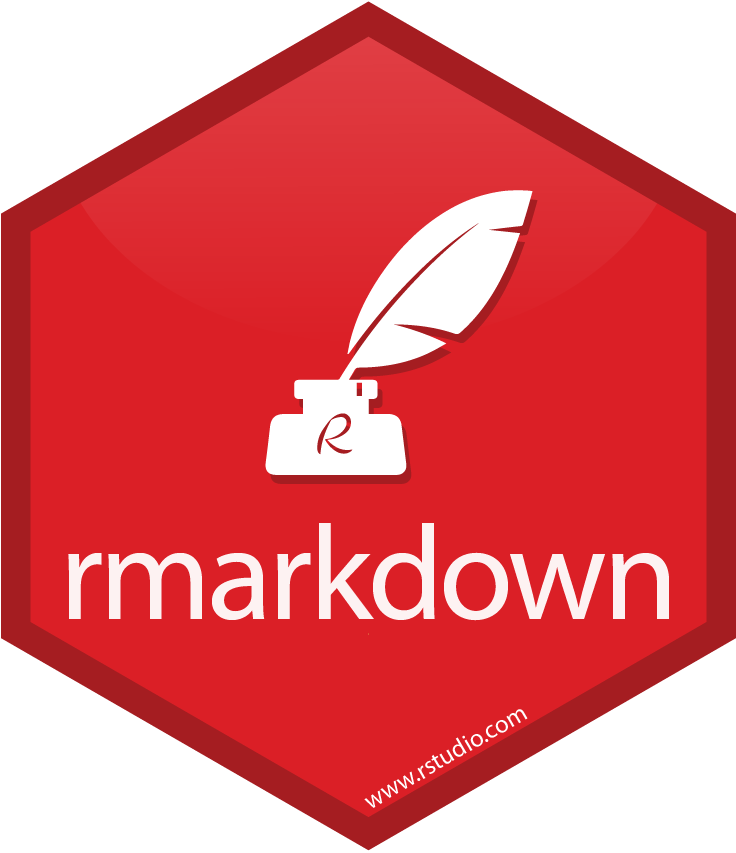Computational notebooks like Jupyter and R Markdown improve:

• Model explorability and interactivity
• Reproducibility

However:

• Exposition is often lacking
• Code may be hard to understand

### The challenge of fragmentation¶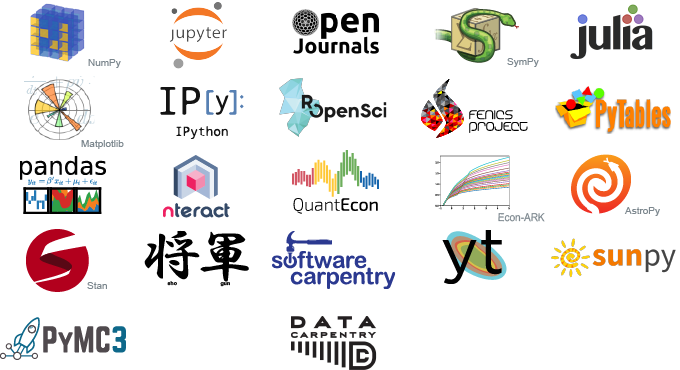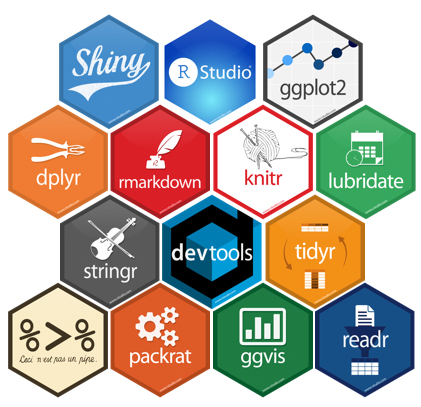Understanding the code is hard because data science is a big tent, encompassing different:

• disciplines
• statistics and machine learning
• software and data engineering
• special sciences: biomedicine, physics, ...
• programming languages
• general: Python, R, Julia, ...
• DSLs: BUGS, Stan, ...
• frameworks and packages
• PyData: pandas, sklearn, statsmodels, ...
• Base R and the tidyverse
• Deep learning: TensorFlow, Caffe, Torch, ...

## Communicating data analysis, the future¶

Open source and open science are producing ever more data science code.
But once it is published, how do we utilize it?

To help people share the results of data analysis, and machines process it, we need a model of data science code that is

• machine-interpretable
• language- and library-agnostic
• not too hard to generate

Goal: Create semantic models of data science code, with minimal human intervention.

## Plan of talk¶

1. Examples
2. Methodology in brief
1. Program analysis
2. Semantic enrichment
3. Future directions

## Example #1: Toy code¶

#### k-means clustering using NumPy and SciPy¶

import numpy as np
from scipy.cluster.vq import kmeans2

iris = np.genfromtxt('iris.csv', dtype='f8', delimiter=',', skip_header=1)
iris = np.delete(iris, 4, axis=1)

centroids, clusters = kmeans2(iris, 3)


#### k-means clustering using pandas and scikit-learn¶

import pandas as pd
from sklearn.cluster import KMeans

iris = iris.drop('Species', 1)

kmeans = KMeans(n_clusters=3).fit(iris.values)
centroids = kmeans.cluster_centers_
clusters = kmeans.labels_


## Example #1: Toy analysis¶

#### k-means clustering in R¶

iris = read.csv("datasets/iris.csv", stringsAsFactors=FALSE)
iris = iris[, names(iris) != "Species"]

km = kmeans(iris, 3)
centroids = km$centers clusters = km$cluster


#### How are the three scripts related?¶

• Different syntactically: different programming languages, different packages
• Equivalent semantically

### Example #1: Semantic flow graph for all three scripts¶## Example #2: DREAM Challenge¶

A real data analysis from the DREAM Challenge for rheumatoid arthritis (RA):

• Question: how well do clinical and genetic covariates predict RA patient response to treatment?
• Process: Contestants submit two models:
1. With clinical covariates only
2. With both clinical and genetic covariates
• Finding: Genetic covariates don't meaningfully increase predictive power

We analyze one top-ranking submission, written in R.

### Example #2: R code¶

library("caret")
library("VIF")
library("Cubist")

merge.p.with.template <- function(p){
template$row = 1:nrow(template) template = template[,c(1,3)] ids = data.resp$IID[is.na(y)]
p = merge(template, p)
p = p[order(p$row), ] p[,c(1,3)] } data = readRDS("pred.rds") resp = readRDS("resp.rds") # non-clinical model data.resp = merge(data, resp[c("FID", "IID", "Response.deltaDAS")]) y = data.resp$Response.deltaDAS
y.training = y[!is.na(y)]

data.resp2 = data.resp[!(names(data.resp) %in% c("Response.deltaDAS", "FID", "IID"))]
dummy = predict(dummyVars(~., data=data.resp2), newdata=data.resp2)

dummy.training = dummy[!is.na(y),]
dummy.testing = dummy[is.na(y),]

v = vif(y.training, dummy.training, dw=5, w0=5, trace=F)
dummy.training.selected = as.data.frame(dummy.training[,v$select]) dummy.testing.selected = as.data.frame(dummy.testing[,v$select])

m1 = cubist(dummy.training.selected, y.training, committees=100)
p1 = predict(m1, newdata=dummy.testing.selected)

# clinical model
dummy = data.resp[c("baselineDAS", "Drug", "Age", "Gender", "Mtx")]
dummy = predict(dummyVars(~., data=dummy), newdata=dummy)
dummy.training = dummy[!is.na(y),]
dummy.testing = dummy[is.na(y), ]

m2 = cubist(dummy.training, y.training, committees=100)
p2 = predict(m2, newdata=dummy.testing)

## create csv files
p1.df = merge.p.with.template(p1)
p2.df = merge.p.with.template(p2)

write.csv(p1.df, quote=F, row.names=F, file="clinical_and_genetic.csv")
write.csv(p2.df, quote=F, row.names=F, file="clinical_only.csv")


### Example #2: Semantic flow graph¶## Methodology in a nutshell¶

How we construct a semantic model of data science code:

Step 1. Dataflow analysis using computer program analysis:

Computer program → raw flow graph (will see soon)

Step 2. Semantic enrichment using knowledge-based methods:

Raw flow graph → semantic flow graph (what we just saw)

### Architecture¶## Program analysis¶

• Uses both static and dynamic analysis
• Records raw flow graph, where
• nodes represent function calls
• edges represent objects and their types

Programming model: "Everything that happens [in R] is a function call" (John Chambers)

### Example #1: Raw flow graphs¶

#### k-means clustering in Python using SciPy (left) and scikit-learn (right)¶### Example #1: Raw flow graphs¶

#### k-means clustering in R¶### Program analysis, step 1: Static analysis¶

In fact, not everything that happens is actually a function call, especially in Python.

In static phase, transform the abstract syntax tree (AST):

1. Homogenize code to conform with programming model:
• Python: transform variable read/assigns, attribute getter/setters, etc. into function calls
• R: handle non-standard evaluation
• All languages: handle mutations
2. Insert callbacks to trace function calls and arguments
• Python: more reliable than sys.settrace
• R: only supports statistical profiling

### Program analysis, step 2: Dynamic analysis¶

In dynamic phase, record the raw flow graph by executing the transformed code:

• Graph built in real-time as code runs
• Provenance record must be maintained

Assumption: Data analysis must be executable: need code, data, and environment,
the same requirement for reproducibility.

## Semantic enrichment¶

Problem: Determine the "meaning" (semantics) of data science code.

Simply impossible without restriction of scope.

Assumption: Code uses semantically meaningful classes and functions from standard packages.

Strategy: Express standard classes and functions in terms of universal concepts.

### Data Science Ontology¶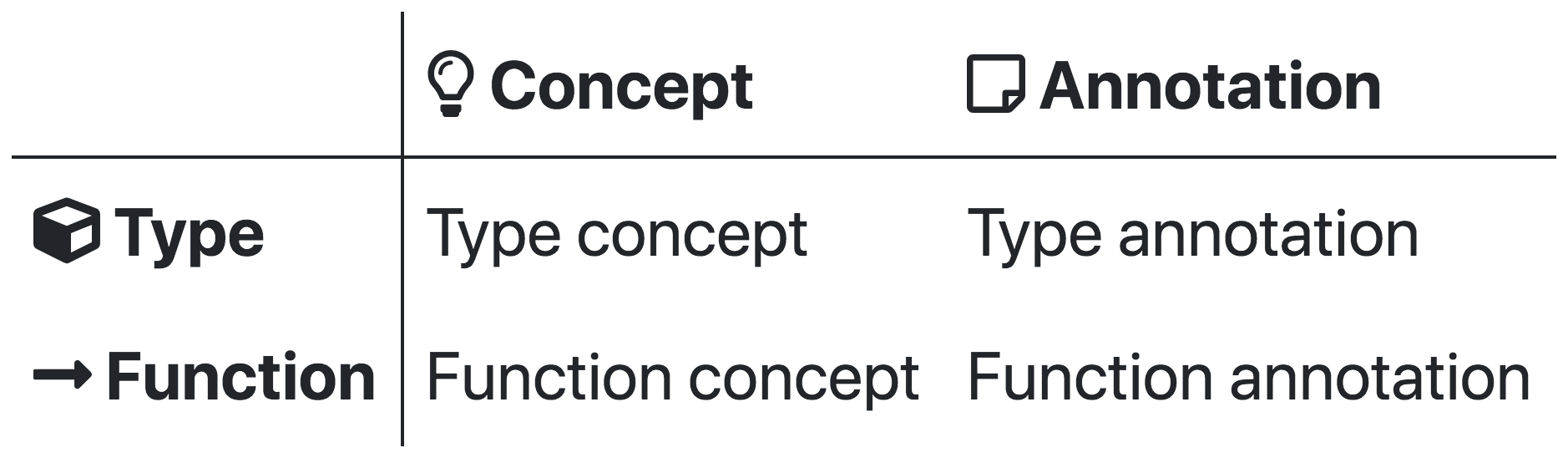• We are building an ontology comprised of:
• concepts from data science
• annotations mapping code to concepts
• Both come in two flavors:
• types (things that exist)
• functions (things that happen)

### Demo: Data Science Ontology¶

#### Example concepts¶k-means clusteringfit supervised model

#### Example annotations¶k-means clustering in scikit-learnk-means clustering in SciPy

### Semantic enrichment algorithm¶

Assumption: Some code will be unannotated, hence semantics will be partial.

Transform raw flow graph to semantic flow graph:

1. Expansion: replace annotated parts with abstract definitions
2. Contraction: simplify unannotated parts as much as possible

Architecture: Implemented in Julia, not Python or R, partly for maximal decoupling.

## Future directions¶

• Improve robustness of program analysis
• Python
• R
• Expand the Data Science Ontology
• Manually
• Partial automation, especially for annotations
• Develop user-facing applications and frontends
• Represent internal mathematical structure of models (current work)

# Thank you!¶

### Contributing¶evan@epatters.orgepattersejpatters